# Difference between revisions of "Smallest quadratic nonresidue is less than square root plus one"

## Statement

Supposeis an odd prime. Supposeis the smallest quadratic nonresidue modulo:is the smallest positive integer less thanthat is a quadratic nonresidue modulo. Then:.

Note that we need theadditive correction term. For instance, in the cases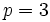and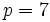, the smallest quadratic nonresidues (andrespectively) are greater than the square root.

In fact, since the smallest quadratic nonresidue modulomust be prime, we conclude that there is a prime less than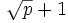that is a quadratic nonresidue modulo.

## Related facts

### Other related facts and conjectures

• Extended Riemann hypothesis: A stronger form of the Riemann hypothesis that is equivalent to the statement that the smallest quadratic nonresidue modulo, for any odd prime, is less than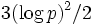, wherehere is the natural logarithm.
• Artin's conjecture on primitive roots: This is a closely related conjecture that states that every integer that is notor a perfect square occurs as a primitive root for infinitely many primes.

## Proof

Given: An odd prime,is the smallest positive integer that is a quadratic nonresidue modulo.

To prove:.

Proof: Letbe the smallest positive integer such that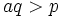, and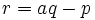. Sinceis prime,, so. Further, by the multiplicativity of Legendre symbols: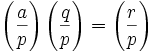.

Sinceis a quadratic nonresidue mod, the first term is, so at least one ofandis a nonresidue. Sinceandis the least nonresidue by assumption,must be a quadratic residue, forcingto be a quadratic nonresidue, and hence,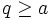. Since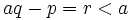, we get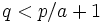. In particular, since, from which we can deduce that.